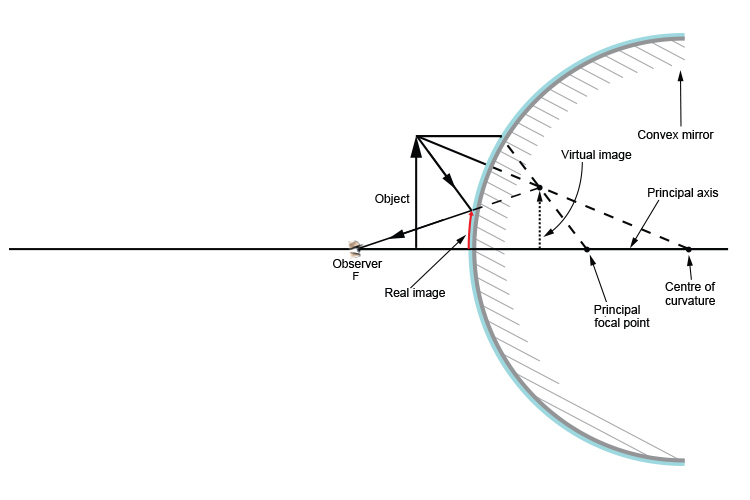# Moving the object distance

So far we have shown what happens when we move the observer but we now need to show you what happens if we keep the observer stationary but move the object.

## Object at more than 2F but less than infinity

Below is the ray diagram for an object which is more than two principal focal lengths away from the convex mirror but less than infinity (infinity being a place that is so far away it can't be reached).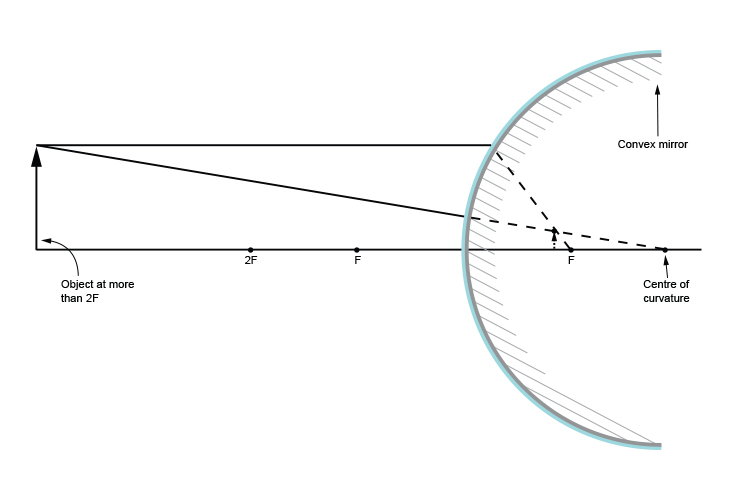Now we shall draw the same diagram but we will include the incident and reflected ray when the observer is at one principal focal length away.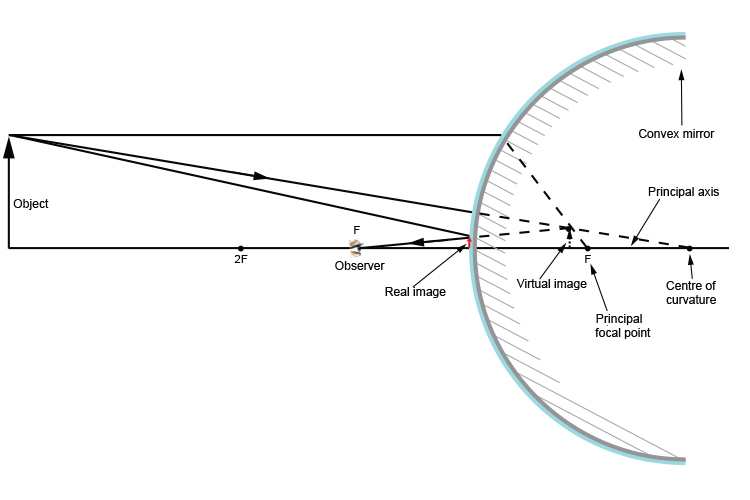## Object at 2F

Below is the ray diagram for an object which is at two principal focal lengths away from the convex mirror.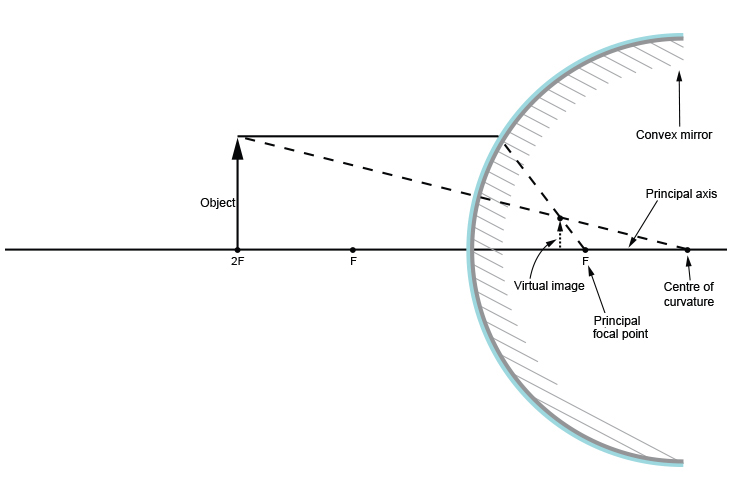Now we shall draw the same diagram but we will include the incident and reflected ray when the observer is at one principal focal length away.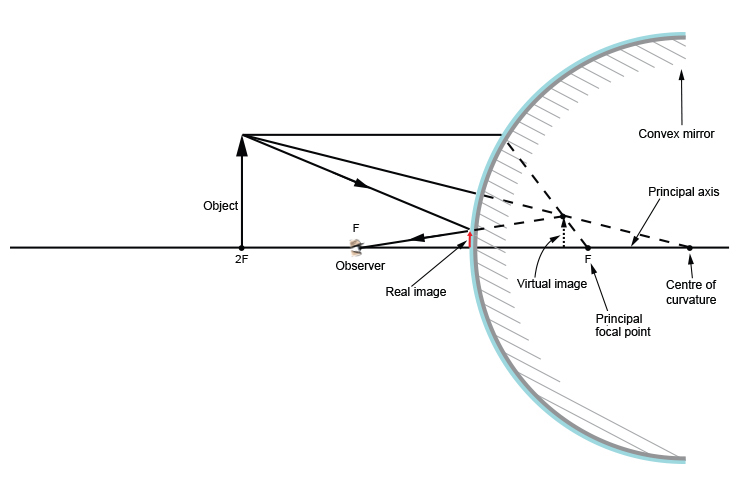## Object between 2F and F

Below is the ray diagram for an object which is between two principal focal lengths and one focal length away from the convex mirror.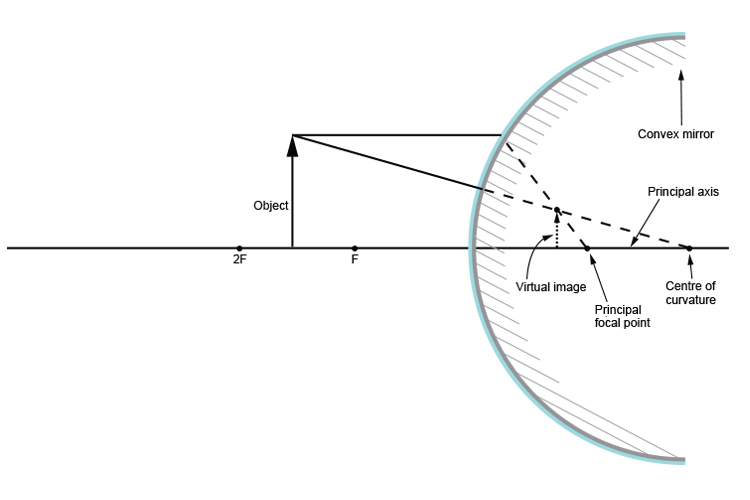Now we shall draw the same diagram but we will include the incident and reflected ray when the observer is at one principal focal length away.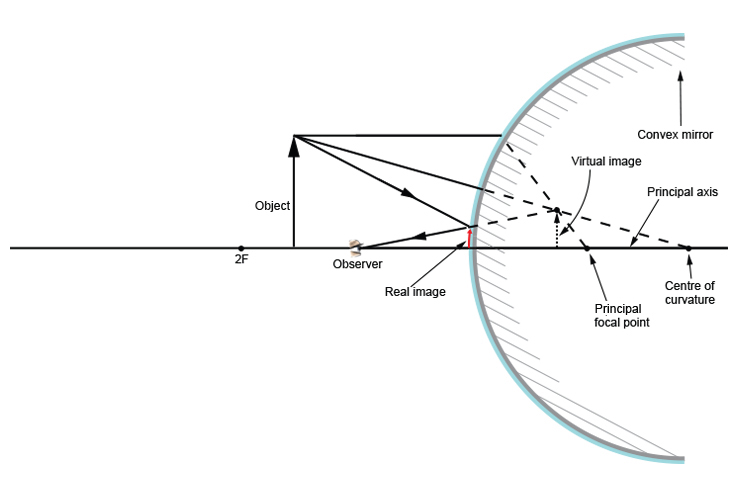## Object at F

Below is the ray diagram for an object which is one principal focal length away from the convex mirror.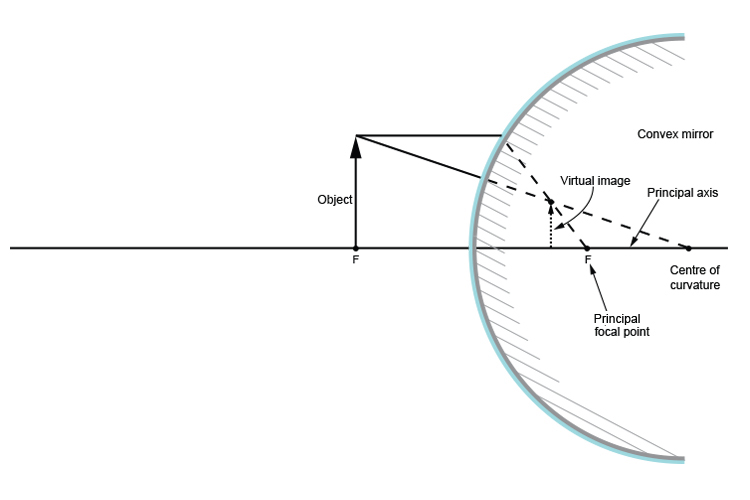Now we shall draw the same diagram but we will include the incident and reflected ray when the observer is at one principal focal length away.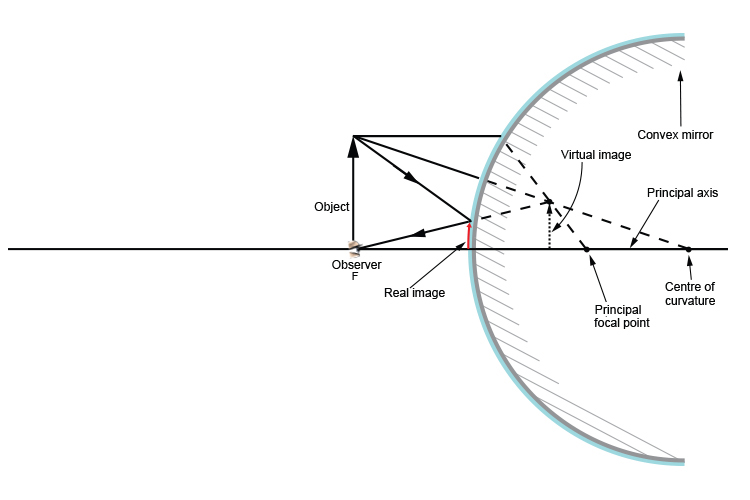## Object between the convex mirror and F

Below is the ray diagram for an object which is between the convex mirror and one principal focal length away.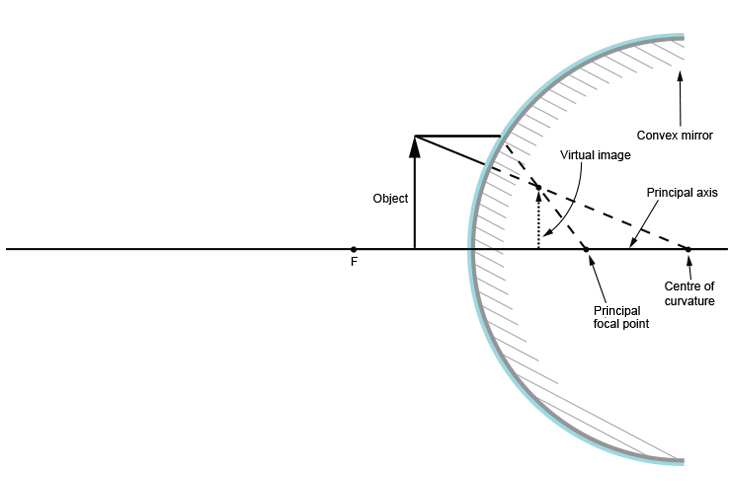Now we shall draw the same diagram but we will include the incident and reflected ray when the observer is at one principal focal length away.